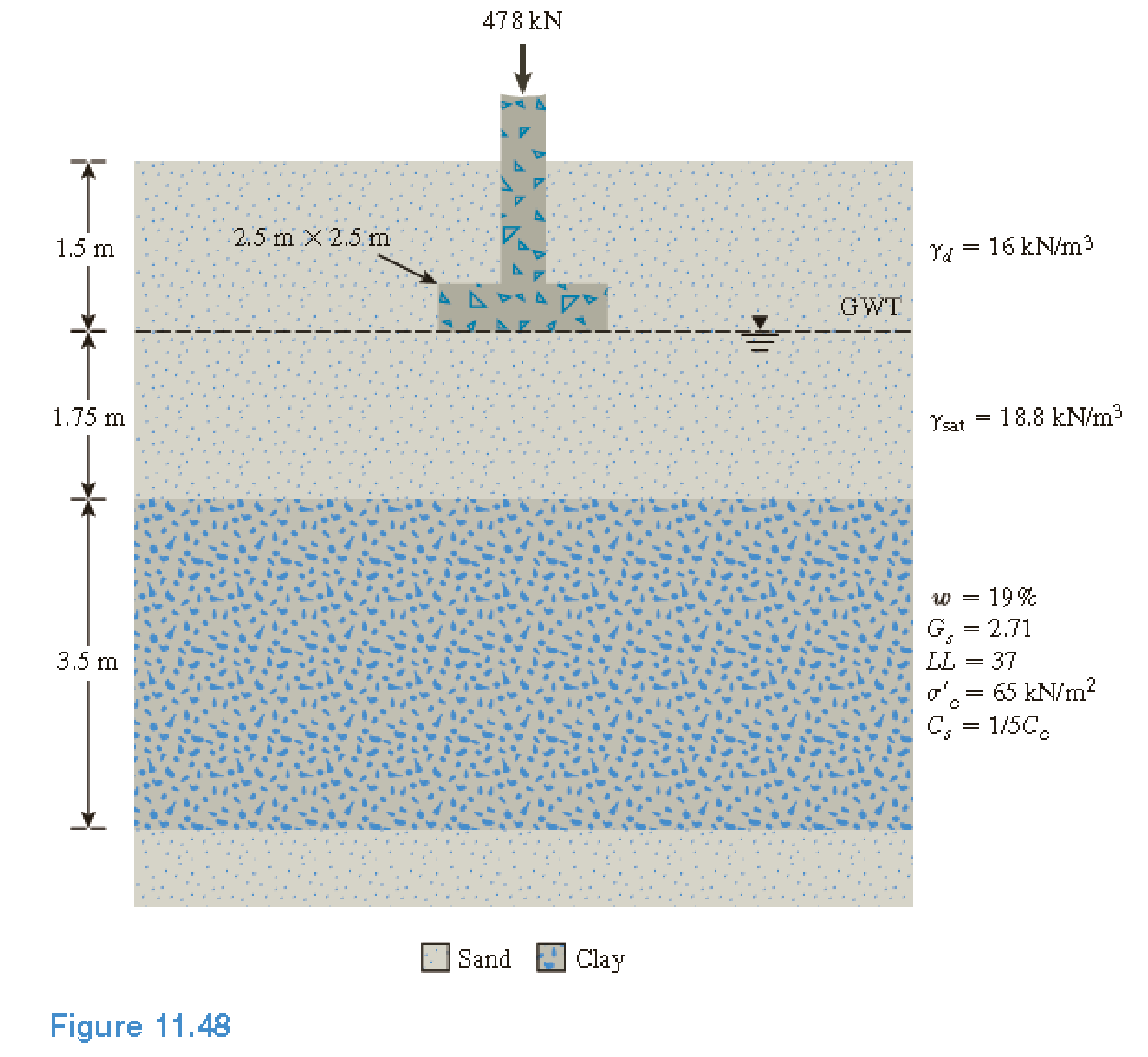Chapter 11, Problem 11.21PPrinciples of Geotechnical Enginee...

9th Edition
Braja M. Das + 1 other
ISBN: 9781305970939

Solutions

Chapter
SectionPrinciples of Geotechnical Enginee...

9th Edition
Braja M. Das + 1 other
ISBN: 9781305970939
Textbook Problem

Refer to figure 11.48. A square foundation, 2.5 × 2.5 m in size, supports a column load of 478 kN. The soil characteristics are given in the figure. Field monitoring indicated that the foundation settlement was 46 mm at the end of 2 years. a. Estimate the average stress increase in the clay layer due to the applied load. b. Estimate the primary consolidation settlement. c. What is the degree of consolidation after 2 years? d. Estimate the coefficient of consolidation for the pressure range. e. Estimate the settlement in 3 years.(a)

To determine

Calculate the average stress increase in the clay layer due to the applied load.

Explanation

Given information:

The foundation load (P) is 478kN.

The length of the foundation (L) is 2.5m.

The breadth of the foundation (B) is 2.5m.

The depth of foundation (Df) is 1.5m.

The height of the clay layer 1 (H1) is 3.25m.

The height of the clay layer 2 (H2) is 3.5m.

The dry unit weight of sand (γd) is 16kN/m3.

The saturated unit weight (γsat) is 18.8kN/m3.

The liquid limit (LL) is 37.

Calculation:

Consider the unit weight of water (γw) is 9.81kN/m3.

Calculate the distributed load (q) as shown below.

q=PBL

Substitute 478kN for P, 2.5m for B, and 2.5m for L.

q=4782.5×2.5=4786.25=76.48kN/m2

Calculate the increase in vertical stress (Δσz) below the center of a rectangular area using the relation as follows.

Δσz=qI4 (1)

Here, I4 is the influence factor and as a function of m1 and n1.

For the depth (z) of 1.75m:

Calculate the width (b) as shown below.

b=B2

Substitute 2.5m for B.

b=2.52=1.25m

Calculate the ratio (m1) as shown below.

m1=LB

Substitute 2.5m for L and 2.5m for B.

m1=2.52.5=1

Calculate the ratio (n1) as shown below.

n1=zb

Substitute 1.25m for b and 1.75m for z.

n1=1.751.25=1

(b)

To determine

Calculate the primary consolidation settlement.

(c)

To determine

Calculate the degree of consolidation after 2 years.

(d)

To determine

Calculate the coefficient of consolidation for the pressure range.

(e)

To determine

Calculate the settlement in 3 years.

Still sussing out bartleby?

Check out a sample textbook solution.

See a sample solution

The Solution to Your Study Problems

Bartleby provides explanations to thousands of textbook problems written by our experts, many with advanced degrees!

Get Started

What is structural independence, and why is it important?

Database Systems: Design, Implementation, & Management

What are three types of cylindrical grinders?

Precision Machining Technology (MindTap Course List)

A typical normal oxygen sensor signal will toggle between and volts.

Automotive Technology: A Systems Approach (MindTap Course List)

What is strategy?

Management Of Information Security

What do the letters UL signify?

EBK ELECTRICAL WIRING RESIDENTIAL

Explain the relationship between logical and physical design.

Systems Analysis and Design (Shelly Cashman Series) (MindTap Course List)

Which type of light is the most likely to cause burns? Why?

Welding: Principles and Applications (MindTap Course List)

A(n) _____ is a request for specific information from a database.

Enhanced Discovering Computers 2017 (Shelly Cashman Series) (MindTap Course List)